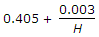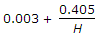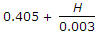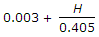# Mechanical Engineering - Hydraulics and Fluid Mechanics

### Exercise :: Hydraulics and Fluid Mechanics - Section 5

6.

The vacuum pressure can be measured with the help of a piezometer tube.

 A. True B. False

Answer: Option B

Explanation:

No answer description available for this question. Let us discuss.

7.

A jet of water discharging from a 40 mm diameter orifice has a diameter of 32 mm at its vena contracta. The coefficient of contraction is

 A. 0.46 B. 0.64 C. 0.78 D. 0.87

Answer: Option B

Explanation:

No answer description available for this question. Let us discuss.

8.

According to Bazin's formula, the discharge over a rectangular weir is mL2g x H3/2 where m is equal to

 A.B.C.D.Answer: Option A

Explanation:

No answer description available for this question. Let us discuss.

9.

A vertical wall is subjected to a pressure due to one kind of liquid, on one of its sides. Which of the following statement is correct?

 A. The pressure on the wall at the liquid level is minimum. B. The pressure on the bottom of the wall is maximum. C. The pressure on the wall at the liquid level is zero, and on the bottom of the wall is maximum D. The pressure on the bottom of the wall is zero.

Answer: Option C

Explanation:

No answer description available for this question. Let us discuss.

10.

Water is a __________ fluid.

 A. real B. ideal C. newtonian D. non-newtonian

Answer: Option C

Explanation:

No answer description available for this question. Let us discuss.

#### Current Affairs 2021

Interview Questions and Answers